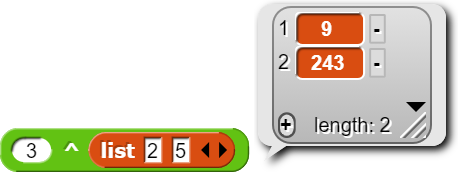# How do you reverse the order of numbers in exponentiation

...without changing the result? I know you can do it for commutative operations like addition and multiplication:

$$3+5=5+3$$
$$3\times5=3\times3$$

and you can, with a different approach, do the same with division and subtraction:

$$3-5=-5+3$$
$$3\div5=$$ $$1\over5$$ $$\times\space3$$

Is there a similar way to rearrange exponentiation ( $$3^5$$ )?

Without changing the result? No.

[sound ot trumpets] The Fundamental Theorem of Arithmetic states that every positive integer has a unique prime factorization.

So, a positive integer that's a product of five threes can't possibly equal a number that's a product of fives.

I mean, yeah, you can do $$(5^{\log_5 3})^{({3^{\log_3 5})}}$$ but I'm guessing you'll agree that that's cheating.

The reason is that I want to add Point arithmetic to my Snap testing, and if I have, say, 3^(point x: (2) y: (5)), I want to have it return (point x: (3^2) y: (3^5)).

Oh. So you don't want to commute two operands; you want to distribute over three of them.

I'm not sure what you want taking a number to a Point power to mean. If those are points on the complex plane, so x is the real part and y is the imaginary part, then
$$a^{x+iy} = a^x \cdot a^{i y} = a^x \cdot (\cos y + i \sin y)$$. If not, I'm not sure how to interpret a number raised to a point power — not just what the formula is, but what you want it to mean.

I pretty much want it to mean something a lot like taking a number to the power of a list. (A list of two numbers can actually be used as a point in regular Snap!, but I want points to be more explicitly made)

Oh. As you say, you can already do that with hyperblocks. Do you have an application in mind that'd use it?

(I'm not sure I was clear about what I mean by "mean." For example, if you think of a list of numbers as representing a vector, then 3 × [4, 5, 6] (scalar times vector) is the operation of scaling (a/k/a zoom, in computer contexts). But [1, 2, 3] × [4, 5, 6] as done by hyperblocks is mathematically meaningless. (There are two mathematical ways to multiply two vectors. One of them, the dot product vw, is a single number, the sum (COMBINE WITH +) of the items of the hyperblock product. The other, the cross product v×w, is more complicated and is a vector perpendicular to the plane containing v and w.) The details aren't immediately relevant; the point is that we have a story — well, two stories — to tell about what it means to multiply two vectors. I don't know of a story about taking a number to the power of a point.)

I've wanted to know how to implement that for a while.

What "it"? Points? Taking a number to a Point power?

https://www.mathsisfun.com/algebra/vectors-cross-product.html

Probably

What does this mean?

That was two questions, not one.

Ah. But for $$a$$ and $$b$$ I only have 2D vectors, so $$c$$, the cross product, would have an x coordinate of $$a_y0-0b_y=0-0=0$$ and a y coordinate of $$0b_x-a_x0=0-0=0$$, so the cross product of two 2D vectors would be $$(0,0,a_xb_y-a_yb_x)$$, which could be represented as a single number.

However, I have no idea how to implement it for an arbitrary number of vectors.Yes, but I want to, in the code, do something to the Point, asking it for $$(x^a,y^a)$$. Because how would a JS number know what it means to raise it to the power of a Point?

Taking a number to a Point power. (I may be dumb but I do know what points are good for!)

I'm pretty sure you can't have a cross product without three dimensions.

Ah, I finally understand why you started this thread asking about reversing the order. You want to say point.NumberToThePowerOfMe(number). You could literally do that, inventing points as objects; see Section VIII of the manual.

But I still don't understand why you think that'd be meaningful and/or useful!

Ah. In that case, I don't know.

Yes, but you can have 2D vectors in 3D, it's just that the z coordinates are 0.

Someone long before me invented Points.

I'm doing it in the JavaScript code, the source code of Snap! testing.

Yes, and if you take the cross product of two 2D vectors in 3D space that are in the xy plane, the result will be a vector pointing parallel to the z axis, perpendicular to the xy plane.

Ah, but you'll learn more if you do it entirely in Snap!.

:~D